A mass m slips along the wall of a semispherical surface of radius R. The velocity at the bottom of the surface is [ MP PMT 1993]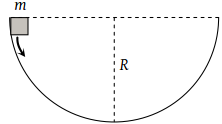(1) $\sqrt{Rg}$

(2) $\sqrt{2Rg}$

(3) $2\sqrt{\pi Rg}$

(4) $\sqrt{\pi Rg}$

Concept Questions :-

Gravitational Potential energy
High Yielding Test Series + Question Bank - NEET 2020

Difficulty Level:

Three different objects of masses and m3 are allowed to fall from rest and from the same point ‘O’ along three different frictionless paths. The speeds of the three objects, on reaching the ground, will be in the ratio of

(1) ${m}_{1}:{m}_{2}:{m}_{3}$

(2) ${m}_{1}:2{m}_{2}:3{m}_{3}$

(3) 1 : 1 : 1

(4) $\frac{1}{{m}_{1}}:\frac{1}{{m}_{2}}:\frac{1}{{m}_{3}}$

Concept Questions :-

Gravitational Potential energy
High Yielding Test Series + Question Bank - NEET 2020

Difficulty Level:

When a body moves with a constant speed along a circle

(1) No work is done on it

(2) No acceleration is produced in the body

(3) No force acts on the body

(4) Its velocity remains constant

Concept Questions :-

Work done by variable force
High Yielding Test Series + Question Bank - NEET 2020

Difficulty Level:

A sphere of mass m is tied to end of a string of length l and rotated through the other end along a horizontal circular path with speed v. The work done by centripetal force in full horizontal circle is

(1) 0

(2) $\left(\frac{m{v}^{2}}{l}\right) . 2\pi l$

(3) $\text{mg\hspace{0.17em}.\hspace{0.17em}2πl}$

(4) $\left(\frac{m{v}^{2}}{l}\right) . \left(l\right)$

Concept Questions :-

Work done by variable force
High Yielding Test Series + Question Bank - NEET 2020

Difficulty Level:

A ball is suspended by a thread of length l. What minimum horizontal velocity has to be imparted to the ball for it to reach the height of the suspension:

(1) gl

(2) 2 gl

(3) $\sqrt{gl}$

(4) $\sqrt{2gl}$

Concept Questions :-

Work-Energy theorem
High Yielding Test Series + Question Bank - NEET 2020

Difficulty Level:

A body of mass m hangs at one end of a string of length l, the other end of which is fixed. It is given a horizontal velocity so that the string would just reach where it makes an angle of 60° with the vertical. The tension in the string at mean position is

(1) 2 mg

(2) mg

(3) 3 mg

(4) $\sqrt{3}mg$

Concept Questions :-

Uniform circular motion
High Yielding Test Series + Question Bank - NEET 2020

Difficulty Level:

As per given figure to complete the circular loop what should be the radius if initial height is 5?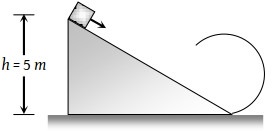(1) 4 m

(2) 3 m

(3) 2.5 m

(4) 2 m

Concept Questions :-

Work-Energy theorem
High Yielding Test Series + Question Bank - NEET 2020

Difficulty Level:

The kinetic energy k of a particle moving along a circle of radius R depends on the distance covered s as k = as2 where a is a constant. The force acting on the particle is

(1) $2a\frac{{s}^{2}}{R}$

(2) $2as{\left(1+\frac{{s}^{2}}{{R}^{2}}\right)}^{1/2}$

(3) 2 as

(4) $2a\frac{{R}^{2}}{s}$

High Yielding Test Series + Question Bank - NEET 2020

Difficulty Level:

A stone of mass 1 kg tied to a light inextensible string of length $L=\frac{10}{3}m$ is whirling in a circular path of radius L in a vertical plane. If the ratio of the maximum tension in the string to the minimum tension in the string is 4 and if g is taken to be 10 m/sec2, the speed of the stone at the highest point of the circle is

(1) 20 m/sec

(2) $10\sqrt{3}m/\mathrm{sec}$

(3) $5\sqrt{2}\text{\hspace{0.17em}}m/\mathrm{sec}$

(4) 10 m/sec

Concept Questions :-

Work-Energy theorem
High Yielding Test Series + Question Bank - NEET 2020

Difficulty Level:

A small block is shot into each of the four tracks as shown below. Each of the tracks rises to the same height. The speed with which the block enters the track is the same in all cases. At the highest point of the track, the normal reaction is maximum in:

 (1)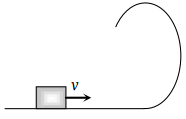(2)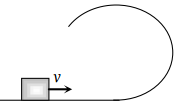(3)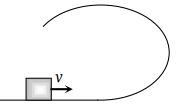(4) Same in all cases

Concept Questions :-

Work-Energy theorem